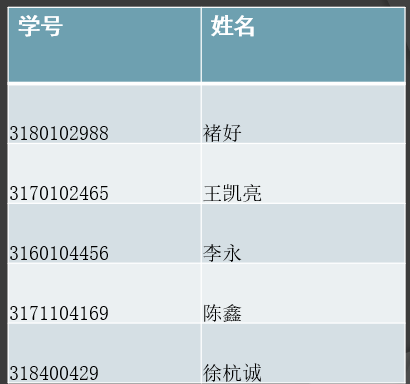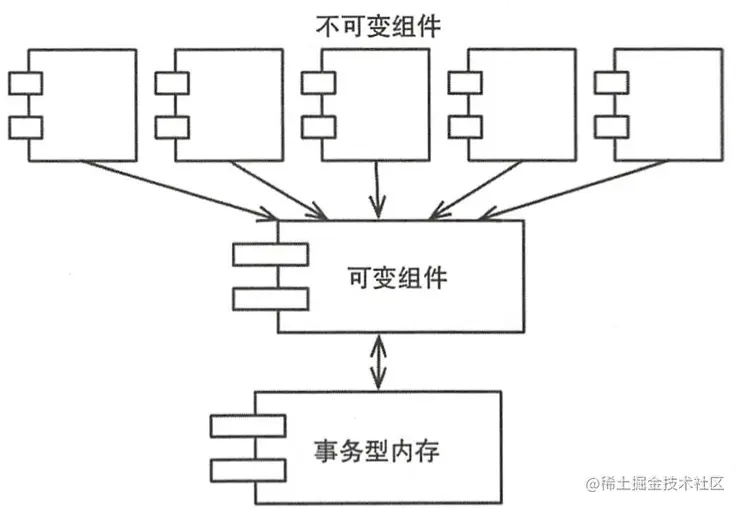# 函数式思维和函数式编程

+关注继续查看

quicksort :: Ord a => [a] -> [a]
quicksort [] = []
quicksort (p:xs) =
(quicksort lesser) ++ [p] ++ (quicksort greater)
where
lesser = filter (< p) xs
greater = filter (>= p) xs

p。然后对这两组数据递归的使用这种算法。

public class Quicksort  {
private int[] numbers;
private int number;

public void sort(int[] values) {
if (values == null || values.length == 0){
return;
}
this.numbers = values;
number = values.length;
quicksort(0, number - 1);
}

private void quicksort(int low, int high) {
int i = low, j = high;
int pivot = numbers[low + (high-low)/2];

while (i <= j) {
while (numbers[i] < pivot) {
i++;
}
while (numbers[j] > pivot) {
j--;
}

if (i <= j) {
swap(i, j);
i++;
j--;
}
}
if (low < j)
quicksort(low, j);
if (i < high)
quicksort(i, high);
}

private void swap(int i, int j) {
int temp = numbers[i];
numbers[i] = numbers[j];
numbers[j] = temp;
}
}

JAVA:

public class Quicksort {
private int[] numbers;

public void sort(int[] values) {

}
}
HASKELL:

quicksort :: Ord a => [a] -> [a]
JAVA:

if (values.length == 0 || values == null) {
return;
}
HASKELL:

quicksort [] = []
JAVA:

public void sort(int[] values) {
if (values.length == 0 || values == null) {
return;
}
this.numbers = values;
this.length = values.length;
quicksort(0, length - 1);
}
HASKELL:

No code. Nothing. Nada. That's good.
JAVA:

p的list)。每次在i方向遍历中发现有比j方向遍历的当前值大时，交互它们的位置。当i的位置超过j时，停止比较，对形成的两个新队列继续递归调用。

private void quicksort(int low, int high) {
int i = low, j = high;
int pivot = numbers[low];

while (i <= j) {
while (numbers[i] < pivot) {
i++;
}
while (numbers[j] > pivot) {
j--;
}

if (i <= j) {
swap(i, j);
i++;
j--;
}
}

if (low < j)
quicksort(low, j);
if (i < high)
quicksort(i, high);
}

private void swap(int i, int j) {
int temp = numbers[i];
numbers[i] = numbers[j];
numbers[j] = temp;
}

quicksort (p:xs) = (quicksort lesser) ++ [p] ++ (quicksort greater)

    where
lesser = filter (< p) xs
greater = filter (>= p) xs

public List<Comparable> sort(List<Comparable> elements) {
if (elements.size() == 0) return elements;

Stream<Comparable> lesser = elements.stream()
.filter(x -> x.compareTo(pivot) < 0)
.collect(Collectors.toList());

Stream<Comparable> greater = elements.stream()
.filter(x -> x.compareTo(pivot) >= 0)
.collect(Collectors.toList());

List<Comparable> sorted = new ArrayList<Comparable>();

return sorted;

}

 Haskell from scratch courtesy of “Learn you a Haskell for Great Good!”

 This quicksort in Haskell that I am showing here is not in-place quicksort so it loses one of its properties, which is memory efficiency. The in-place version in Haskell would be more like:

import qualified Data.Vector.Generic as V
import qualified Data.Vector.Generic.Mutable as M

qsort :: (V.Vector v a, Ord a) => v a -> v a
qsort = V.modify go where
go xs | M.length xs < 2 = return ()
| otherwise = do
p <- M.read xs (M.length xs div 2)
j <- M.unstablePartition (< p) xs
let (l, pr) = M.splitAt j xs
k <- M.unstablePartition (== p) pr
go l; go \$ M.drop k pr
Discussion here.

 This version of quicksort is simplified for illustration purposes. It’s always good looking at the source. Boldly go and read this piece of History (with a capital H) by C.A.R. Hoare, “Quicksort”.

 Will we consider uncontrolled state harmful the same way GOTO statement being considered harmful consolidated structured programming?

 A big question mark over time on functional programming languages has been the ability (or lack thereof) to effectively code User Interfaces. Don’t despair though! There’s this cool new thing called Functional Reactive Programming (FRP). Still performing babysteps, but there are already implementations out there. One that’s gaining lots of momentum is ReactJS/Om/ClojureScript web app stack. Guess that might be a good follow-up post :)0 00 00 02. 函数式编程

690 01395 0http://www.ruanyifeng.com/blog/2012/04/functional_programming.html
519 0980 01011 0（转）现代C++函数式编程

1084 0

Python语言基础 - 函数、面向对象、异常处理5448753

Java面向对象开发23594106

R语言编程基础6588

JavaScript面向对象的程序设计